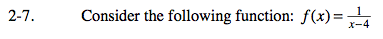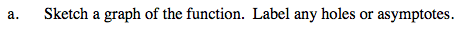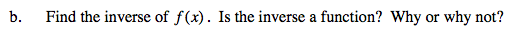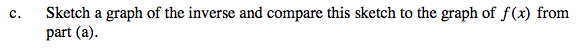### Home > CALC > Chapter Ch2 > Lesson 2.1.1 > Problem2-7

2-7.No holes. Two asymptotes: x = 4 and y = 0. Label them.$y=\frac{1}{x-4}\ \ \ \ \ \text{Rename }f(x)\text{ as }y$

$y(x-4)=1 \ \ \ \text{solve for }x$

$x-4=\frac{1}{y}$

$x=\frac{1}{y}+4$

$f^{-1}(x)=\frac{1}{x}+4 \ \ \ \text{Switch the }x \text{ and }y$You do not need to know the inverse equation to sketch a graph of the inverse. Start by switching the horizontal and vertical asymptotes. Then plot some key points, and connect.

Use the eTool below to explore the graphs.
Click on the link to the right to view the full version of the eTool. Calc 2-7 HW eTool